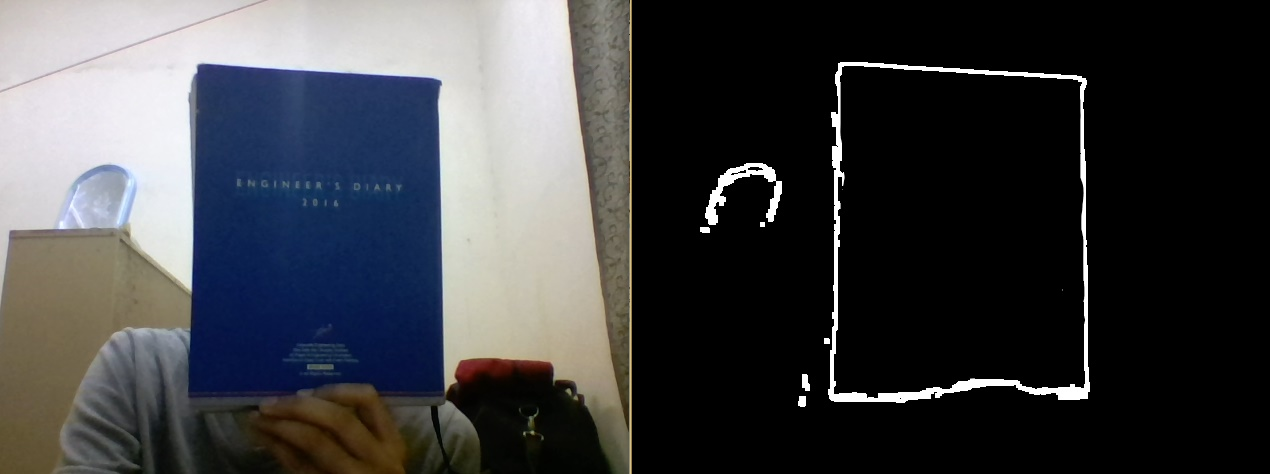# Python | Morphological Operations in Image Processing (Gradient) | Set-3

In the previous articles, the Opening operation and the Closing operations were specified. In this article, another morphological operation is elaborated that is Gradient. It is used for generating the outline of the image. There are two types of gradients, internal and external gradient. The internal gradient enhances the internal boundaries of objects brighter than their background and external boundaries of objects darker than their background. For binary images, the internal gradient generates a mask of the internal boundaries of the foreground image objects.

Parameters:
-> image: Input Image array.
-> kernel: Structuring element.

Below is the Python code explaining Gradient Morphological Operation –

 `# Python programe to illustrate ` `# Gradient morphological operation ` `# on input frames ` ` `  `# organizing imports   ` `import` `cv2   ` `import` `numpy as np   ` ` `  `# return video from the first webcam on your computer.   ` `screenRead ``=` `cv2.VideoCapture(``0``) ` ` `  `# loop runs if capturing has been initialized. ` `while``(``1``): ` `    ``# reads frames from a camera ` `    ``_, image ``=` `screenRead.read() ` `     `  `    ``# Converts to HSV color space, OCV reads colors as BGR  ` `    ``# frame is converted to hsv ` `    ``hsv ``=` `cv2.cvtColor(image, cv2.COLOR_BGR2HSV) ` `     `  `    ``# defining the range of masking ` `    ``blue1 ``=` `np.array([``110``, ``50``, ``50``]) ` `    ``blue2 ``=` `np.array([``130``, ``255``, ``255``]) ` `     `  `    ``# initializing the mask to be ` `    ``# convoluted over input image ` `    ``mask ``=` `cv2.inRange(hsv, blue1, blue2) ` ` `  `    ``# passing the bitwise_and over ` `    ``# each pixel convoluted ` `    ``res ``=` `cv2.bitwise_and(image, image, mask ``=` `mask) ` `     `  `    ``# defining the kernel i.e. Structuring element ` `    ``kernel ``=` `np.ones((``5``, ``5``), np.uint8) ` `     `  `    ``# defining the gradient function  ` `    ``# over the image and structuring element ` `    ``gradient ``=` `cv2.morphologyEx(mask, cv2.MORPH_GRADIENT, kernel) ` `    `  `    ``# The mask and closing operation ` `    ``# is shown in the window  ` `    ``cv2.imshow(``'Gradient'``, gradient) ` `     `  `    ``# Wait for 'a' key to stop the program  ` `    ``if` `cv2.waitKey(``1``) & ``0xFF` `=``=` `ord``(``'a'``): ` `        ``break` ` `  `# De-allocate any associated memory usage   ` `cv2.destroyAllWindows() ` ` `  `# Close the window / Release webcam  ` `screenRead.release() `

Result:The output image frame shows the outline generated over the blue book and the blue object in top left.

My Personal Notes arrow_drop_upCheck out this Author's contributed articles.

If you like GeeksforGeeks and would like to contribute, you can also write an article using contribute.geeksforgeeks.org or mail your article to contribute@geeksforgeeks.org. See your article appearing on the GeeksforGeeks main page and help other Geeks.

Please Improve this article if you find anything incorrect by clicking on the "Improve Article" button below.

Article Tags :

Be the First to upvote.

Please write to us at contribute@geeksforgeeks.org to report any issue with the above content.